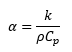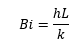you are here:/ News & Insights / Engineering Advantage Blog / Calculating Solution Settings for a Transient Thermal Analysis - Part 1

# Calculating Solution Settings for a Transient Thermal Analysis - Part 1May 10, 2016 By: Eric Stamper

To preface this topic, let's cover a few basics.

Question: In a heat transfer analysis, which material will heat up faster if the thermal conductivity and density properties are nearly the same, a material with a higher or lower specific heat?

Answer: A material with the lower specific heat will heat up faster than a material with a higher specific heat, if the conductivity and density are the same.

The important property that decides how quickly a material will respond to changes in a thermal environment is termed the thermal diffusivity, α, which is the ratio of thermal conductivity to the heat capacity and has units of length2/time.Where: k =thermal conductivity, ρ=density, and Cp=specific heat

This quantity "α" relates the ability of the material to transport thermal energy through conduction (k) relative to its ability to store it (ρ*Cp). Meaning that materials with a high α will respond quickly to thermal environment changes, (i.e. the material is better at conducting thermal energy than storing it) and materials with a low α will respond slowly and take longer to arrive at a new equilibrium. As an example, metals generally have a higher α than nonmetallic materials.

The problem shown below demonstrates this behavior for various materials.

Figure 1 shows a metallic handle surrounded by insulation on all but one end. The metallic body is initially at a uniform temperature (300°K) until the exposed end (x=0) experiences convective heating, causing the body to increase to a new equilibrium temperature (350°K). The problem is treated as one dimensional in x, and the temperature variation in the metal at the insulated end of the body (x=L) throughout time is shown in Figure 2 for various materials. The materials are also listed in the legend in order of descending α (in units of 1e-6m2/s).

Figure 1: Sample Problem

## conduction1.pngFigure 2: Thermal Response of Various Materials

## conduction2.pngThe response of various materials to an environmental change is shown in Figure 2, illustrating the influence of the thermal diffusivity property. However, for conduction problems that involve surface convection, the profile of the temperature distribution along the length at any point in time and ultimately the temperature at the insulated end (x=L)  is also a function of the Biot number, Bi.Where: h=convective heat transfer coefficient, L=characteristic length, and k=thermal conductivity.

The Biot number influences problems that involve surface convection, as it relates the ratio of the temperature drop across the material to the difference between the convection surface temperature and environment. For small Bi numbers, the thermal energy will pass through conduction much easier than it can travel across the fluid boundary layer through convection. As a result, the temperature distribution along the length of the handle at any point in time will be approximately uniform, as shown in Figure 3, for the lowest Bi number. In addition, as the Bi number increases (in this case by increasing the convective film coefficient); the resistance to convection across the surface will be much less than the resistance to conduction within the solid (it will more readily heat up). A higher Bi number will also result in a larger thermal gradient (the difference in temperature between x=0 and x=L) for a transient point in time.

Figure 3: Thermal Gradient in Zinc at 1,000s For Varying Bi Numbers

## conduction3.pngStay tuned for Part 2 of this post, where these properties will be used to calculate the transient thermal finite element analysis solution settings!